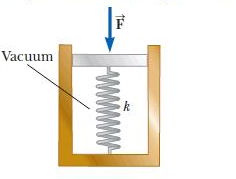# The spring of the pressure gauge shown in the figure below has a force constant of 1400 N/m, and...

## Question:

The spring of the pressure gauge shown in the figure below has a force constant of {eq}1400 \ N m^{-1} {/eq}, and the piston has a diameter of {eq}1.00 \ cm. {/eq} As the gauge is lowered into water in a lake, what change in depth causes the piston to move in by {eq}0.680 \ cm {/eq} ?## Spring Force:

The Spring Force is the force exerted by a spring in order for it to return to its relaxed position. The spring force according to Hooke's law is proportional to the length by which the spring is either stretched or compressed. Each spring also has a unique response by which it can be stretched or compressed described by spring constant, k.

## Answer and Explanation:

Become a Study.com member to unlock this answer! Create your account

Given:

{eq}k = 1400 \frac {N}{m} {/eq} Spring constant

{eq}D = 1.00 \ cm = 0.01 \ m {/eq} Diameter of the piston

{eq}x = 0.680 \ cm = 6.8 \times...

See full answer below.

#### Learn more about this topic:Practice Applying Spring Constant Formulas

from

Chapter 17 / Lesson 11
3.4K

In this lesson, you'll have the chance to practice using the spring constant formula. The lesson includes four problems of medium difficulty involving a variety of real-life applications.